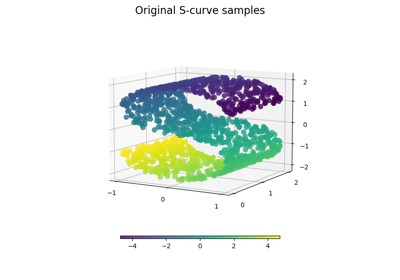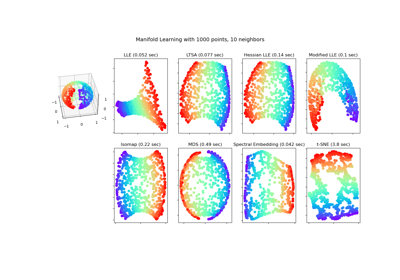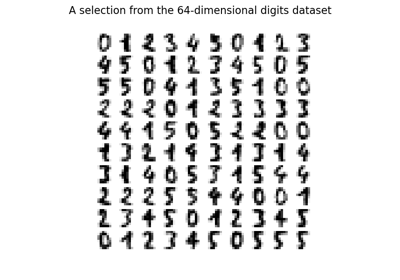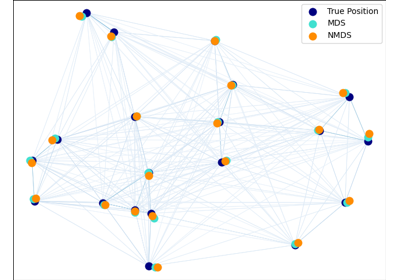# sklearn.manifold.MDS¶

class sklearn.manifold.MDS(n_components=2, *, metric=True, n_init=4, max_iter=300, verbose=0, eps=0.001, n_jobs=None, random_state=None, dissimilarity='euclidean')[source]

Multidimensional scaling.

Read more in the User Guide.

Parameters:
n_componentsint, default=2

Number of dimensions in which to immerse the dissimilarities.

metricbool, default=True

If True, perform metric MDS; otherwise, perform nonmetric MDS. When False (i.e. non-metric MDS), dissimilarities with 0 are considered as missing values.

n_initint, default=4

Number of times the SMACOF algorithm will be run with different initializations. The final results will be the best output of the runs, determined by the run with the smallest final stress.

max_iterint, default=300

Maximum number of iterations of the SMACOF algorithm for a single run.

verboseint, default=0

Level of verbosity.

epsfloat, default=1e-3

Relative tolerance with respect to stress at which to declare convergence.

n_jobsint, default=None

The number of jobs to use for the computation. If multiple initializations are used (n_init), each run of the algorithm is computed in parallel.

None means 1 unless in a joblib.parallel_backend context. -1 means using all processors. See Glossary for more details.

random_stateint, RandomState instance or None, default=None

Determines the random number generator used to initialize the centers. Pass an int for reproducible results across multiple function calls. See Glossary.

dissimilarity{‘euclidean’, ‘precomputed’}, default=’euclidean’

Dissimilarity measure to use:

• ‘euclidean’:

Pairwise Euclidean distances between points in the dataset.

• ‘precomputed’:

Pre-computed dissimilarities are passed directly to fit and fit_transform.

Attributes:
embedding_ndarray of shape (n_samples, n_components)

Stores the position of the dataset in the embedding space.

stress_float

The final value of the stress (sum of squared distance of the disparities and the distances for all constrained points).

dissimilarity_matrix_ndarray of shape (n_samples, n_samples)

Pairwise dissimilarities between the points. Symmetric matrix that:

• either uses a custom dissimilarity matrix by setting dissimilarity to ‘precomputed’;

• or constructs a dissimilarity matrix from data using Euclidean distances.

n_features_in_int

Number of features seen during fit.

New in version 0.24.

feature_names_in_ndarray of shape (n_features_in_,)

Names of features seen during fit. Defined only when X has feature names that are all strings.

New in version 1.0.

n_iter_int

The number of iterations corresponding to the best stress.

sklearn.decomposition.PCA

Principal component analysis that is a linear dimensionality reduction method.

sklearn.decomposition.KernelPCA

Non-linear dimensionality reduction using kernels and PCA.

TSNE

T-distributed Stochastic Neighbor Embedding.

Isomap

Manifold learning based on Isometric Mapping.

LocallyLinearEmbedding

Manifold learning using Locally Linear Embedding.

SpectralEmbedding

Spectral embedding for non-linear dimensionality.

References

“Modern Multidimensional Scaling - Theory and Applications” Borg, I.; Groenen P. Springer Series in Statistics (1997)

“Nonmetric multidimensional scaling: a numerical method” Kruskal, J. Psychometrika, 29 (1964)

“Multidimensional scaling by optimizing goodness of fit to a nonmetric hypothesis” Kruskal, J. Psychometrika, 29, (1964)

Examples

>>> from sklearn.datasets import load_digits
>>> from sklearn.manifold import MDS
>>> X.shape
(1797, 64)
>>> embedding = MDS(n_components=2)
>>> X_transformed = embedding.fit_transform(X[:100])
>>> X_transformed.shape
(100, 2)


Methods

 fit(X[, y, init]) Compute the position of the points in the embedding space. fit_transform(X[, y, init]) Fit the data from X, and returns the embedded coordinates. get_params([deep]) Get parameters for this estimator. set_params(**params) Set the parameters of this estimator.
fit(X, y=None, init=None)[source]

Compute the position of the points in the embedding space.

Parameters:
Xarray-like of shape (n_samples, n_features) or (n_samples, n_samples)

Input data. If dissimilarity=='precomputed', the input should be the dissimilarity matrix.

yIgnored

Not used, present for API consistency by convention.

initndarray of shape (n_samples,), default=None

Starting configuration of the embedding to initialize the SMACOF algorithm. By default, the algorithm is initialized with a randomly chosen array.

Returns:
selfobject

Fitted estimator.

fit_transform(X, y=None, init=None)[source]

Fit the data from X, and returns the embedded coordinates.

Parameters:
Xarray-like of shape (n_samples, n_features) or (n_samples, n_samples)

Input data. If dissimilarity=='precomputed', the input should be the dissimilarity matrix.

yIgnored

Not used, present for API consistency by convention.

initndarray of shape (n_samples, n_components), default=None

Starting configuration of the embedding to initialize the SMACOF algorithm. By default, the algorithm is initialized with a randomly chosen array.

Returns:
X_newndarray of shape (n_samples, n_components)

X transformed in the new space.

get_params(deep=True)[source]

Get parameters for this estimator.

Parameters:
deepbool, default=True

If True, will return the parameters for this estimator and contained subobjects that are estimators.

Returns:
paramsdict

Parameter names mapped to their values.

set_params(**params)[source]

Set the parameters of this estimator.

The method works on simple estimators as well as on nested objects (such as Pipeline). The latter have parameters of the form <component>__<parameter> so that it’s possible to update each component of a nested object.

Parameters:
**paramsdict

Estimator parameters.

Returns:
selfestimator instance

Estimator instance.

## Examples using sklearn.manifold.MDS¶Comparison of Manifold Learning methods

Comparison of Manifold Learning methodsManifold Learning methods on a severed sphere

Manifold Learning methods on a severed sphereManifold learning on handwritten digits: Locally Linear Embedding, Isomap…

Manifold learning on handwritten digits: Locally Linear Embedding, Isomap...Multi-dimensional scaling

Multi-dimensional scaling